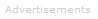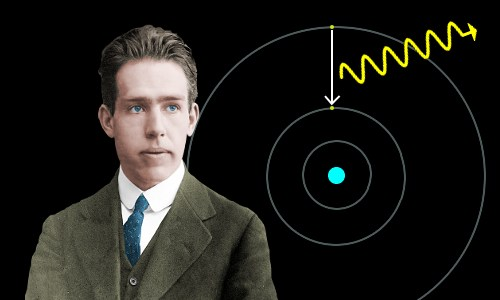# Definition of Electrons

What are Electrons?

The electron is the universe's fundamental unit of negative charge. It is believed to be truly fundamental, because it cannot be split into smaller particles, and it has no detectable structure. Its symbol is usually e- or β-.### Electron Charge

All electrons carry the elementary charge, which is -1.602 x 10-19 C.

### Electric and Magnetic Fields

Like all charges, the electron's charge generates its own electric field.

Since the electron is always in motion, like any moving charge, it generates its own magnetic field.

The atoms of every chemical element have an equal number of negatively charged electrons and positively charged protons - they are therefore electrically neutral.

### Mass

Electrons have a tiny mass: 9.109 x 10-31 kg (to four significant figures).

Even compared with protons, the electron mass is small: the proton:electron mass ratio is 1836 (to four significant figures).

### Diameter

The electron is believed to have no diameter and no volume. It is thought to be a point particle.

### Location and Space Occupied

In atoms, electrons occupy a volume of space centered on the nucleus. Electrons move within a much larger volume than the nucleus occupies.

• For example, a hydrogen atom's nucleus (a single proton) has a diameter of 1.75 x 10-15 m.
• The Bohr diameter of the hydrogen atom, mainly electron orbital space, is 1.06 x 10-10 m.

The difference between these diameters is a factor of about 60,000.

### Electrons and Compounds

Electron energy levels and their interactions determine the chemical behavior and bonding of substances. Common examples of this are:

• atoms may ionize to form compounds
• atoms may form compounds by sharing electrons in covalent bonds

### Beta Particles

During radioactive beta-decay, a neutron decays into a proton. This is accompanied by the ejection from the nucleus of an electron antineutrino and a very high energy electron known as a beta particle, symbol β-.

### Electricity and Magnetism

Static electricity results from the movement of electrons from one body on to another: this separation of electric charge means one body is positively charged and the other negatively charged.

Electric current is the flow of electric charge - usually electrons - although it could be ions. Electric conductivity is based on the ease of movement of electrons.

Magnetism results from the movement of electrons.

### Heat

Electrons play a major role in thermal conduction.

### The Need for Quantum Physics

In atoms, electrons are bound to the nucleus by electrostatic attraction. In classical physics, electrons should lose energy and spiral into the positive nucleus. If this were the case, atoms would have a very limited lifetime. Classical physics, therefore, cannot explain the existence of atoms.

In quantum physics, electrons may only occupy certain defined energy levels and, under normal conditions, will not combine with the protons in the nucleus. (Electrons do actually combine with protons in the extreme conditions associated with neutron stars.)Niels Bohr built the first quantum mechanical model of the atom, which demanded that electrons occupy definite orbits. Light is emitted or absorbed when an electron jumps between levels.

### Wave-Particle Duality

Quantum physics established the principle of wave-particle duality or the matter wave: particles may behave like waves with velocities, wavelengths, amplitudes, and frequencies; and can be reflected, refracted and diffracted.

### The de Broglie Equation and Electron Waves

The wavelength of matter waves can be calculated from the de Broglie equation:

λ = h / p

where: λ = wavelength; h = Planck's constant; and p = momentum.

Electrons have wavelengths and can behave like waves.

### The Schrödinger Equation

The Schrödinger equation allows an electron's quantum mechanical wave function, Ψ, to be calculated.

With Ψ and Ψ2 we get three of the four quantum numbers that characterize electrons in an atom or molecule and the shapes and orientations of electron orbitals.

### The Dirac Equation and Electron Spin

The Dirac equation, a wave equation for electrons travelling at speeds close to the speed of light, naturally produces the quantum mechanical property of electron spin.

An electron can have spin of either + ½ or - ½. Subatomic particles which can have spin of + ½ or - ½ are called fermions. Unlike bosons, no two fermions can occupy the same quantum state, so they are subject to the Pauli exclusion principle.

Electrons are fermions and obey the Pauli exclusion principle.

### Quantum Numbers

Every electron in an atom is characterized by four quantum numbers: n, l, ml, and ms.

• n: the principal quantum number
• l: the orbital angular momentum quantum number
• ml: the magnetic quantum number
• ms: the spin quantum number

The Pauli exclusion principle says that no two electrons in an atom can have the same four quantum numbers: every electron must exist in a unique state. Electrons in different atoms fulfil this demand, because the atoms are in different locations in space.

### Angular Momentum

In addition to mass and charge, electrons possess angular momentum. This comes in two forms: orbital angular momentum associated with the electron orbiting the nucleus; and spin angular momentum, mentioned above.

### Electron Diffraction

Electron diffraction methods for determining the atomic and molecular structures of solids rely on the wave behavior of electrons.

### Electromagnetism

Photons of visible light are produced or absorbed when electrons jump between allowed energy levels within atoms.Search the Dictionary for More Terms